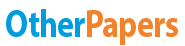# Power System Economics

Essay by   •  May 17, 2017  •  Case Study  •  270 Words (2 Pages)  •  757 Views

## Essay Preview: Power System Economics

Report this essay
Page 1 of 2

[pic 1]

Princess Sumaya University for Technology

King Abdullah II Faculty for Engineering

Power System Economics

 Student Name ID Ahmad Hanbali 20140030 Omar Hisham 20120018

MATLAB:

After opening the MATLAB software, we copied the code that was given to us previously, and then we edited the parameters in that code of the cost equation as follows:

[pic 2]

The next step was running the MATLAB program. The value of lambda that was entered is 13, and an iterative method solved the equations as follows:

[pic 3]

The graph is shown below:

[pic 4]

Power World Simulator:

We ran the PWS software, then we opened the file case of example 12.8.

Then we solved the system using economic dispatch and got the following results:

[pic 5]

The IC-Curve for the system is:

[pic 6]

Conclusion:

We can clearly notice that there's a difference between the results we got from the two soft-wares.

The first obvious difference is the marginal cost, which is 5724.14 \$/hr in PWS software and is 4694\$/hr in the MATLAB . The reason for that in the MATLAB software we neglected the transmission losses, that was not done in the PWS software.

The second one is that the PWS software is more user-friendly showing the system elements, while in the MATLAB the user is only dealing with a code.

The next difference is that the MATLAB software is more flexible in changing the axis division than the PSW software.

One major difference is that the MATLAB software uses iterative method unlike the PWS software, which uses the analytical method to solve for lambda.

There was slightly difference in the value of lambda between both soft-wares .

On the other hand, both soft-wares gave the same generation power for each plant.

...

...